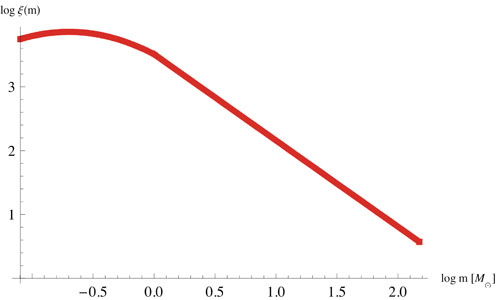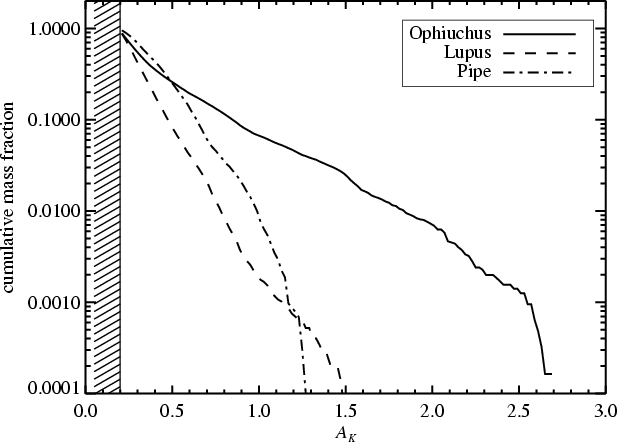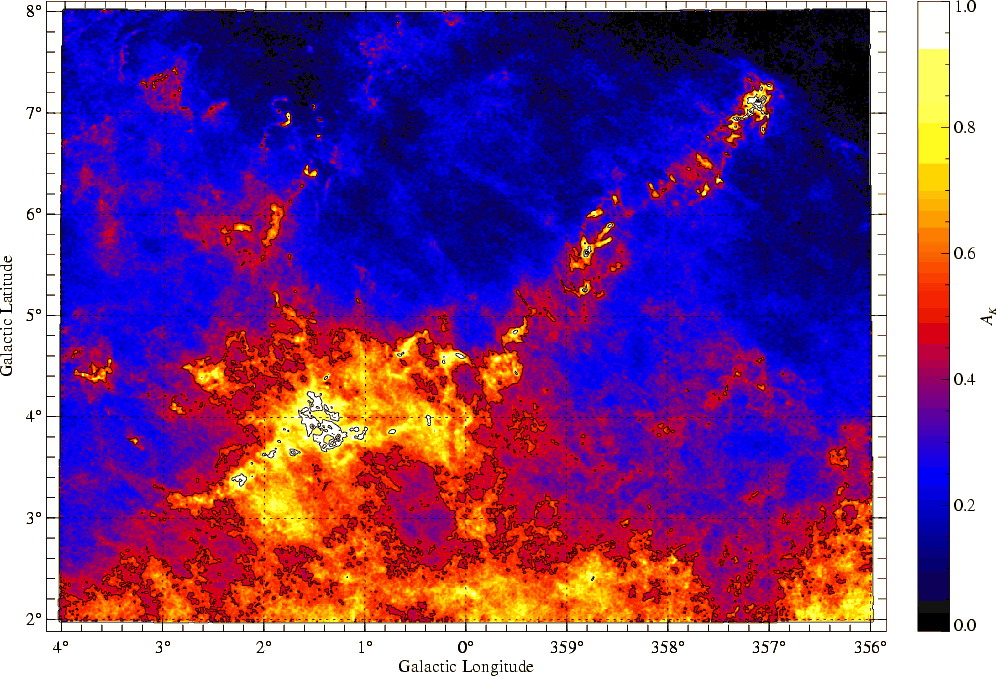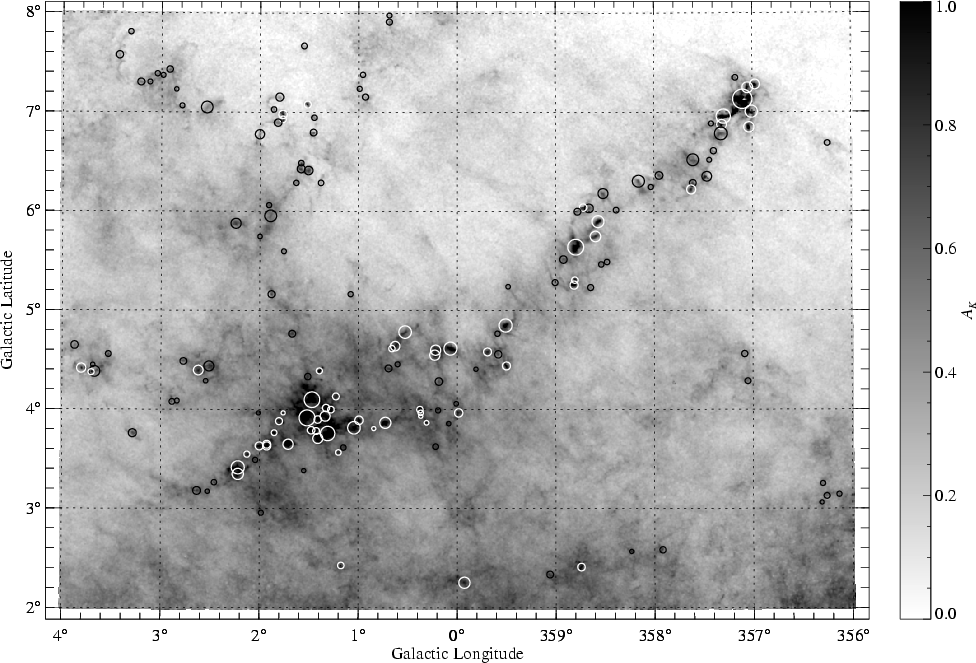Harvard Astronomy 201b

ARTICLE: The mass function of dense molecular cores and the origin of the IMF (2007)

In Journal Club, Journal Club 2013, Uncategorized on March 12, 2013 at 9:30 pm

The mass function of dense molecular cores and the origin of the IMF

by  Joao Alves Marco Lombardi & Charles Lada
Summary by Doug Ferrer

Introduction

One of the main goals of researching the ISM is understanding the connection between the number and properties of stars and the properties of the surrounding galaxy. We want to be able to look at a stellar population and deduce what sort of material it came from, and the reverse–predict what sort of stars we should expect to form in some region given what we know about its properties. The basics of this connection have been known for a while (eg. Bok 1977). Stars form from the gravitational collapse of dense cores of molecular clouds. Thus the properties of stars are the properties of these dense cores modulated by the physical processes that happen during this collapse.

One of the key items we would like to be able to derive from this understanding of star formation is the stellar initial mass function (IMF)–the number of stars of a particular mass as a function of mass. Understanding how the IMF varies from region to region and across time would be extremely useful in many areas of astrophysics, from cosmology to star clusters. In this paper, Alves et al. attempt to explain the origin of the IMF and provide evidence for this explanation by examining the molecular cloud complex in the Pipe nebula. We will look at some background on the IMF, then review the methods used by Alves et al. and asses the implications for star formation.

The IMF and its Origins

The IMF describes the probability density for any given star to have a mass M, or equivalently the number of stars in a given region with a mass of M. Early work done by Salpeter (1955) showed that the IMF for relatively high mass stars ($M > 1 M_{sun}$) follows a power law with the index$\alpha \approx -1.3 - 1.4$. For lower masses, the current consensus is for a break below$1 M_{sun}$, and another break below$.1 M_{sun}$ , with a peak around$.3 M_{sun}$. A cartoon of this sort of IMF is shown in Fig. 1.  The actual underlying distribution may in fact be log-normal. This is consistent with stars being formed from collapsing over-densities caused by supersonic turbulence within molecular clouds (Krumholz 2011). This is not particularly strong evidence, however, as the log-normal distribution can result from any process that depends on the product of many random variables.Figure 1: An illustration of the accepted form of the IMF. There is a power law region for less than$1 M_{sun}$, and a broad peak for lower masses. Image from Krumholz (2011) produced using Chabrier (2003)

The important implication of these results is that there is a characteristic mass scale for star formation of around$.2-.3 M_{sun}$ . There are two (obvious) ways to explain this:

1. The efficiency of star formation peaks at certain scales.
2. There is a characteristic scale for the clouds that stars form from

There has been quite a lot of theoretical work examining option 1 (see Krumhoz 2011 for a relatively recent, accessible review). There are many different physical processes at play in star formation–turbulent MHD, chemistry, thermodynamics, radiative transfer, and gravitational collapse.  Many of these processes are not separately well understood, and each is occurring in its complex, and highly non-linear regime.  We are not even close to a complete description of the full problem. Thus it would not be at all surprising if there were some mass scale, or even several mass scales that are singled out by the star formation process, even if none is presently known. There is some approximate analytical work showing that feedback from stellar outflows may provide such a scale (eg. Shu et al. 1987) . More recent work (e.g. Price and Bate 2008) has shown that magnetic fields cause significant effects on the structure of collapsing cloud cores in numerical simulations, and may reduce or enhance fragmentation depending on magnetic field strength and mass scale.

Nevertheless, the authors are skeptical of the idea that star-formation is not a scale-free process. Per Larson (2005), they do not believe that turbulence or magnetic fields are likely to be very important for the smallest, densest scale clouds that starts ultimately form from. Supersonic turbulence is quickly dissipated on these scales, and magnetic fields are dissipated through ambipolar diffusion–the decoupling of neutral molecules from the ionic plasma. Thus Larson argues that thermal support is the most important process in small cores, and the Jeans analysis will be approximately correct.

The authors thus turn to option 2. It is clear that if the dense cores of star forming clouds already follow a distribution like the IMF, then there will be no need for option 1 as an explanation. Unfortunately though, the molecular cloud mass function (Fig. 2) does not at first glance show any breaks at low mass and has too shallow of a power law index .  But what if we look at only the smallest, densest clumps?Figure 2: The cumulative cloud mass function (Ak is proortional to mass) for several different cloud complexes from Lombardi et al. (2008). While this is not directly comparable to the IMF, the important take away is that there are no breaks at low mass.

Observations

Observations using dense gas emission tracers like$C^{18} O$ and$HC^{13}O$ produces mass distributions more like the stellar IMF (eg. Tachihara et al. 2002, Onishi 2002) . However, there are many systematic uncertainties in emission based analysis. To deal with these issues, the authors instead probed dense cloud mass using wide field extinction mapping (this work).   An extinction map was constructed of the nearby Pipe nebula using the NICER method of Lombardi & Alves (2001), which we have discussed in class. This produced the extinction map shown in Fig. 3 below.Figure 3: NICER Extinction map of the Pipe nebula. A few dense regions are visible, but the noisey, variable background makes it difficult to seperate out seperate cores in a consistent way.

Data Analysis

The NICER map of the pipe nebula reveals a complex filamentary structure with very high dynamic range in column density ($~ 10^{21} - 10^{22.5} cm^{-2}$). It is difficult to assign cores to regions in such a data set in a coherent way (Alves et al. 2007) using standard techniques–how do we determine what is a core and what is substructure to a core? Since it is precisely the number and distribution of cores that we are interested in, we cannot use a biased method to identify the cores. To avoid this, the authors used a technique called  multiscale wavelet decomposition. Since the authors do not give much information on how this works, we will give a brief overview following a description of a similar technique from Portier-Fozzani et al. (2001).

Wavelet Decomposition

Wavelet analysis is a hybrid of Fourier and coordinate space techniques. A wavelet is a function that  has a characteristic frequency, position and spatial extent, like the one in Fig.  4. Thus if we convolve a wavelet of a given frequency and length with a signal, it will tell us how the spatial frequency of the signal varies with position. This is the type of analysis used to produce a spectrogram in audio visualization.Figure 4: A wavelet. This is one is the product of a sinusoid with a gausian envelope. This choice is a perfect tradeoff between spatial and frequency resolution, but other wavelets may be ideal for some other resolution goal. Note that$\Delta x \Delta k \ge 1/2$

The authors used this technique to separate out dense structures from the background. They performed the analysis using wavelets with spatial scales close to three different length scales separated by factors of 2 (.08 pc, .15 pc, .3 pc). Then they combined the structures identified at each length scale into trees based on spatial overlap, and called only the top level of these trees separate cores. The resulting identified cores are shown in fig. 5.  While the cores are shown as circles in fig. 5, this method does not assume spherical symmetry, and the actual identified core shapes are filamentary, reflecting the qualitative appearance of the imageFigure 5: Dense cores identified in the Pipe nebula (circles) from Alves et al. (2007). The radii of the circles is proportional to the core radius determined by the wavelet decomposition analysis

Results

After obtaining what they claim to be a mostly complete sample of cores, the authors calculate the mass distribution for them. This is done by converting dust extinction to a dust column density. This gives a dust mass for each clump, which can then be extrapolated to a total mass by assuming a set dust fraction.  The result of this is shown in fig. 6 below. The core mass function they obtain is qualitatively similar in shape to the IMF, but scaled by a factor of ~4 in mass. The analysis is only qualitative, and no statistics are done or functions fit to the data. The authors claim that this result evinces a universal star formation efficiency of ~30%, and that this is good agreement with that calculated analytically by (Shu 2004) and numerical simulations. This is again only a qualitative similarity, however.

We should also note that the IMF is hypothesized to be a log-normal distribution. This sort of distribution can arise out of any process that depends multiplicatively on many independent random factors. Thus the fact that dense cores have a mass function that is a scaled version of the IMF is not necessarily good evidence that they share a simple causal link, in the same way that two variables both being normally distributed does not mean that they are any way related.Figure 6: The mass function of dense molecular cores (points) and the IMF (solid grey line). The dotted gray line is the IMF with mass argument scaled by a factor of 4. The authors note the qualitative agreement, but do not perform any detailed analysis.

Conclusions

Based on this, the authors conclude that there is no present need for a favored mass scale in star formation as an explanation of the IMF.  Everything can be explained by the distribution produced by dense cloud cores as they are collapsing.  There are a few points of caution however. This is a study of only one cluster, and the data are analyzed using an opaque algorithm that is not publicly available. Additionally, the distributions are not compared statistically (such as with KS), so we have only qualitative similarity.  It would be interesting to see these results replicated for a different cluster using a more transparent statistical method

References

Bok, B. J., Sim, M. E., & Hawarden, T. G. 1977, Nature, 266, 145

Krumholz, M. R. 2011, American Institute of Physics Conference Series, 1386, 9

Lombardi, M., Lada, C. J., & Alves, J. 2008, A&A, 489, 143

Krumholz, M. R., & Tan, J. C. 2007, ApJ, 654, 304

Alves, J., Lombardi, M., & Lada, C. J. 2007, A&A, 462, L17

Lombardi, M., Alves, J., & Lada, C. J. 2006, A&A, 454, 781

Larson, R. B. 2005, MNRAS, 359, 211

Shu, F. H., Li, Z.-Y., & Allen, A. 2004, Star Formation in the Interstellar Medium: In Honor of
David Hollenbach, 323, 37

Onishi, T., Mizuno, A., Kawamura, A., Tachihara, K., & Fukui, Y. 2002, ApJ, 575, 950

Tachihara, K., Onishi, T., Mizuno, A., & Fukui, Y. 2002, A&A, 385, 909

Portier-Fozzani, F., Vandame, B., Bijaoui, A., Maucherat, A. J., & EIT Team 2001, Sol. Phys.,
201, 271

Shu, F. H., Adams, F. C., & Lizano, S. 1987, ARA&A, 25, 23

Salpeter, E. E. 1955, ApJ, 121, 161